# Volume Of A Cone Worksheet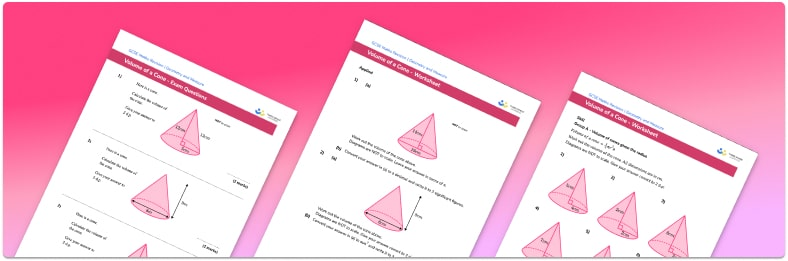• Section 1 of the volume of a cone printable worksheet contains 27 skills-based volume of a cone questions, in 3 groups to support differentiation
• Section 2 contains 4 applied volume of a cone questions with a mix of real-life word problems and deeper problem solving questions
• Section 3 contains 3 foundation and higher level GCSE exam style volume of a cone questions
• An answer key and a mark scheme for all volume of a cone questions are provided
• Questions follow variation theory with plenty of opportunities for students to work independently at their own level
• All questions created by fully qualified expert secondary maths teachers

Suitable for GCSE maths revision for AQA, OCR and Edexcel exam boards

• This field is for validation purposes and should be left unchanged.

You can unsubscribe at any time (each email we send will contain an easy way to unsubscribe). To find out more about how we use your data, see our privacy policy.

### Volume of a cone at a glance

Volume is a measure of how much space there is inside a solid object. To calculate the volume of a cone, we use the volume formula V=(1/3)π(r^2)h where h is the vertical height of the cone and r is the base radius of the cone.

Care should be taken not to confuse the vertical height with the slant height of the cone, which is used to calculate the curved surface area of the cone.

The volume of the cone is measured in units cubed, for example cm^3  or mm^3 . We will often be asked to either give an answer in terms of π or to round the answer to a given degree of accuracy, such as to the nearest tenth.

Looking forward, students can then progress to additional volume worksheets and other geometry worksheets, for example a simplifying expressions worksheet or simultaneous equations worksheet.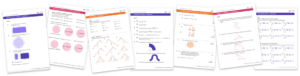For more teaching and learning support on Geometry our GCSE maths lessons provide step by step support for all GCSE maths concepts.

## Related worksheets

Volume Of A Cuboid Worksheet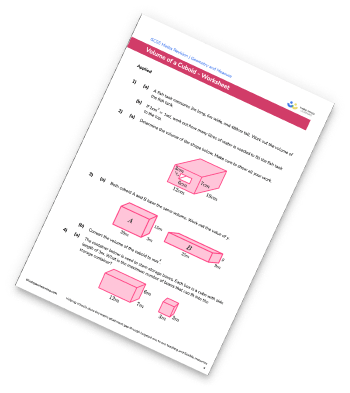Volume Of A Cylinder Worksheet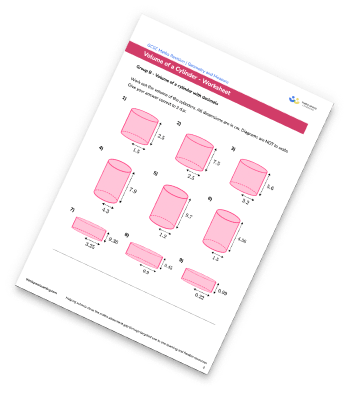Congruent Triangles Worksheet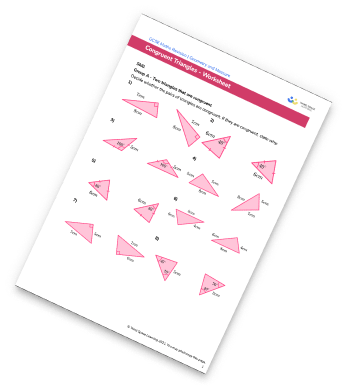Surface Area Of A Triangular Prism Worksheet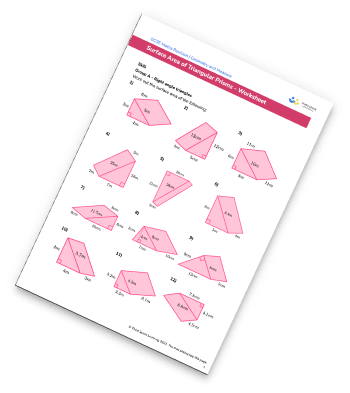## Do you have KS4 students who need more focused attention to succeed at GCSE?There will be students in your class who require individual attention to help them succeed in their maths GCSEs. In a class of 30, it’s not always easy to provide.

Help your students feel confident with exam-style questions and the strategies they’ll need to answer them correctly with our dedicated GCSE maths revision programme.

Lessons are selected to provide support where each student needs it most, and specially-trained GCSE maths tutors adapt the pitch and pace of each lesson. This ensures a personalised revision programme that raises grades and boosts confidence.

Find out more